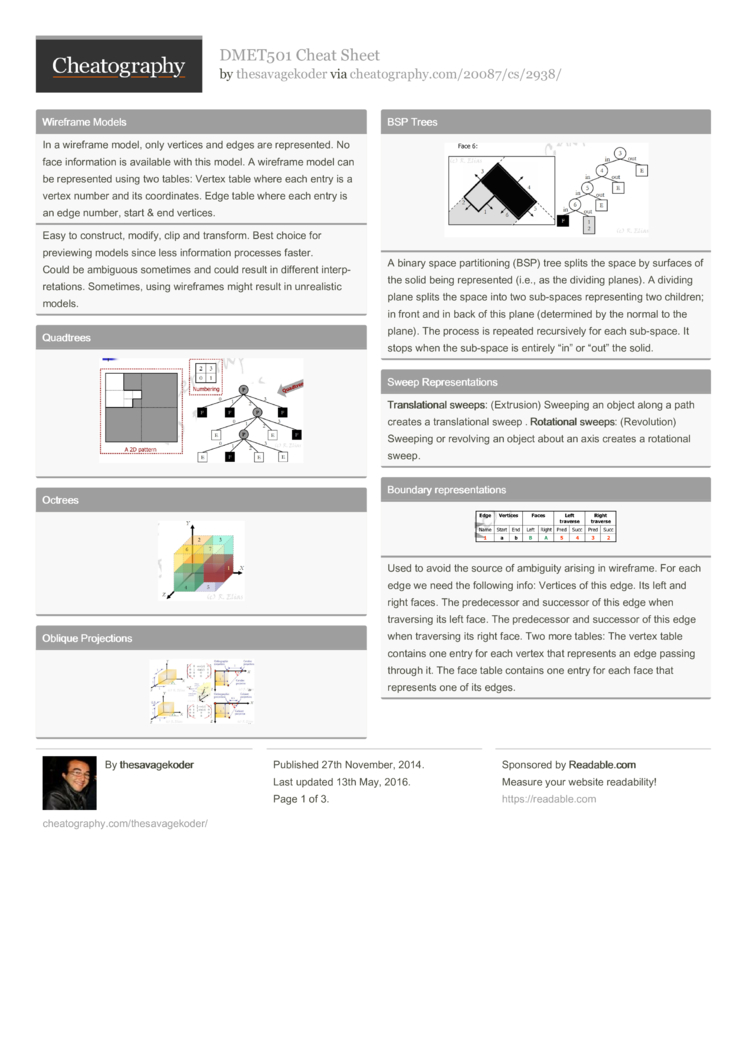# DMET501 Cheat Sheet by thesavagekoder

Solids 3D-Transformations Projections

### Wireframe Models

 In a wireframe model, only vertices and edges are repres­ented. No face inform­ation is available with this model. A wireframe model can be repres­ented using two tables: Vertex table where each entry is a vertex number and its coordi­nates. Edge table where each entry is an edge number, start & end vertices.
Easy to construct, modify, clip and transform. Best choice for previewing models since less inform­ation processes faster.
Could be ambiguous sometimes and could result in different interp­ret­ations. Sometimes, using wireframes might result in unreal­istic models.### Oblique Projec­tions### BSP TreesA binary space partit­ioning (BSP) tree splits the space by surfaces of the solid being repres­ented (i.e., as the dividing planes). A dividing plane splits the space into two sub-spaces repres­enting two children; in front and in back of this plane (deter­mined by the normal to the plane). The process is repeated recurs­ively for each sub-space. It stops when the sub-space is entirely “in” or “out” the solid.

### Sweep Repres­ent­ations

 Tran­sla­tional sweeps: (Extru­sion) Sweeping an object along a path creates a transl­ational sweep . Rota­tional sweeps: (Revol­ution) Sweeping or revolving an object about an axis creates a rotational sweep.

### Boundary repres­ent­ationsUsed to avoid the source of ambiguity arising in wireframe. For each edge we need the following info: Vertices of this edge. Its left and right faces. The predec­essor and successor of this edge when traversing its left face. The predec­essor and successor of this edge when traversing its right face. Two more tables: The vertex table contains one entry for each vertex that represents an edge passing through it. The face table contains one entry for each face that represents one of its edges.

### 1P Perspe­ctiveCOP@Or­igin, z=d
COP@[0­,0,-d], z = 0
The view plane intersects the z -axis. The normal to the view plane is parallel to the z -axis. Two components of the view plane normal n are zeros. Lines parallel to the view plane will be orthog­rap­hically projected as a multi-view projec­tion. Lines parallel to the 3 rd axis (which is parallel to the view plane
normal) converge to a single vanishing point.

### Interior, Exterior and Closure

 The inte­rior of a solid contains all points inside the solid. The clos­ure of a solid contains the interior points plus the points on the surface of the solid. The exte­rior of a solid contains points that do not belong to the closure.

### Spatial Enumer­ation

 In spatial enumer­ation, the space is split into equal-­sized small volume elements or voxels . A voxel may be vacant or occupied. Any 3D model can be repres­ented as a list of occupied voxels.

### Planar Projec­tions### Multi-view Projec­tions### 2P Perspe­ctiveWhen the center of projection is at the origin and the normal to the view plane makes an angle θ with the yz-plane: the overall two-point perspe­ctive projection process can be achieved in three steps.
1. Rotate through an angle −θ about the y -axis so that the normal to the view plane coincides with the z -axis. 2. Perform a one-point perspe­ctive projec­tion. 3. Rotate back through an angle θ about the y -axis.

### Polygonal Modeling

 Inde­pendent faces: each face is indicated as a sequence of vertex coordi­nates. Vertex and face tables: uses two tables; Vertex table: showing vertex numbers and coordi­nates and Face table: showing faces and their surrou­nding vertex numbers. Adja­cency lists: A face is associated with three lists of adjacent vertices, edges and faces. An edge is ... A vertex is ... Efficient way for traversal at the cost of more storage space. Triangle meshes: Only triangular faces are consid­ered. A vertex may be shared among many faces. For each face, surrou­nding vertices and adjacent faces are stored.
A group of adjacent faces comprises what is called a polygonal mesh.

### Transl­ati­on/­Scaling (h)3D Reflection xy-plane: 3D scaling (1,1,-1) yz: (-1,1,1) xz: (1,-1,1)

### Axonom­etric Projection1. Rotate through an angle −θ about the y -axis. 2. Rotate through an angle φ about the x -axis. 3. Use the front view projection matrix

### 3P Perspe­ctiveWhen the center of projection is at the origin and the normal to the view plane
makes an angle θ with the yz-plane and an angle φ with the zx -plane, the overall three-­point perspe­ctive projection process can be achieved in three steps. 1. Rotate through an angle φ about the x -axis so that the normal to the view plane coincides with the zx -plane. 2. Perform a two-point perspe­ctive projec­tion. 3. Rotate back through an angle −φ about the x -axis.3 Pages
//media.cheatography.com/storage/thumb/thesavagekoder_dmet501.750.jpg

PDF (recommended)